# Lecture 35 Backward Euler and Midpoint Rule¶

In this lecture we will see how changing the choice of discretization points leads to alternative methods, the backward Euler and midpoint rule, that have better stability and convergence rates.

## Euler method revisited¶

Recall the $n \times n+1$ finite-difference matrix

$$D_n \triangleq {1 \over h} \begin{pmatrix} -1 & 1 \cr & -1 & 1 \cr &&\ddots & \ddots \cr &&& -1 & 1\end{pmatrix}$$

This arose by approximating functions using piecewise affine approximation, differentiating, and then evaluating at the $n$ points $x_0+0,x_1+0,\ldots,x_{n-1}+0$, where the $+0$ denotes that it is the limit from the left. We depicted this last lecture:

In :
f=x->cos(20cos(x))
n=4

h=1/n

x=linspace(0.,1.,n+1)
vals=f(x)

plot(x,vals)  # do a piecewise approximation of the data specified by vals;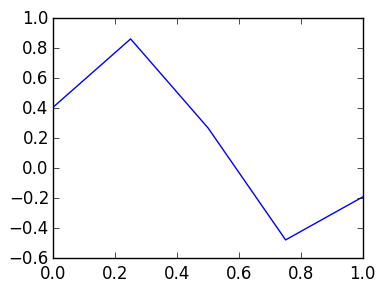In :
#differentiate and evaluate at k*h+0

dvals=diff(vals)*h

for k=1:n
plot([(k-1)*h,k*h],[dvals[k],dvals[k]])
end

scatter(x[1:n],dvals)

axis([0,1,-0.5,0.5]);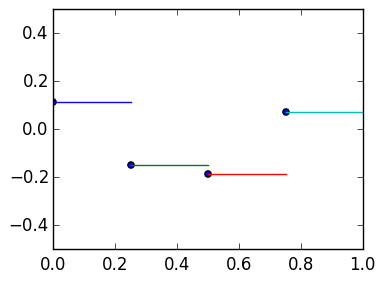Thus we view the matrix $D_n$ as a map from values at $x_0,\ldots,x_n$ to values at $x_0,\ldots,x_{n-1}$, which we denote via:

$$D_n : {\hbox{Values at } \atop x_0,\ldots,x_n} \rightarrow {\hbox{Values at } \atop x_0,\ldots,x_{n-1}}$$

We can create this matrix using

In :
function D(h,n)
ret=zeros(n,n+1)
for k=1:n
ret[k,k]=-1/h
ret[k,k+1]=1/h
end
ret
end

Out:
D (generic function with 1 method)

Indeed, with sufficiently large n, this converges to the true derivative evaluated at $x_0,\ldots,x_{n-1}$:

$$D_n \begin{pmatrix}f(x_0) \cr \vdots \cr f(x_n) \end{pmatrix} \approx \begin{pmatrix} f'(x_0) \cr \vdots \cr f'(x_{n-1}) \end{pmatrix}$$

We verify this:

In :
n=1000
h=1/n
x=0.:h:n*h

f=exp
fp=exp  # derivative of f

Dn=D(h,n)

norm(Dn*f(x)-fp(x[1:end-1]),Inf)

Out:
0.0013582351596626019

The corresponding matrix representing multiplication by $a(x)$ is the $n \times n+1$ matrix

$$A_n \triangleq \begin{pmatrix} a(x_0) \cr & a(x_1) \cr &&\ddots \cr &&&a(x_{n-1}) & 0 \end{pmatrix},$$

where this is a map

$$A_n : {\hbox{Values at } \atop x_0,\ldots,x_n} \rightarrow {\hbox{Values at } \atop x_0,\ldots,x_{n-1}}$$
In :
function A_left(a::Function,h,n)
ret=zeros(n,n+1)
for k=1:n
ret[k,k]=a((k-1)*h)
end
ret
end

Out:
A_left (generic function with 1 method)
In :
a= x-> 1-x.^2
n=10
h=1/n
x=0.:h:n*h

A=A_left(a,h,n)

r=x[1:end-1]

A*f(x)-a(r).*f(r)

Out:
10-element Array{Float64,1}:
0.0
0.0
0.0
-2.22045e-16
0.0
0.0
-2.22045e-16
-2.22045e-16
0.0
0.0        

We finally have the boundary condition corresponding to evaluating at $0$ which is the $1 \times n+1$ row vector

$$B_n = [1,0,\ldots,0]$$

This is a map

$$B_n : {\hbox{Values at } \atop x_0,\ldots,x_n} \rightarrow {\hbox{Value at } \atop x_0}$$

We want to solve

$$u' - a(x)u = f(x) , u(0) = c$$

We do so by replacing the operator $L = D -a(x)$ with its discretization

$$L_n = D_n -A_n$$

which is a map

$$L_n : {\hbox{Values at } \atop x_0,\ldots,x_n} \rightarrow {\hbox{Values at } \atop x_0,\ldots,x_{n-1}}$$

We verify that this converges to the true $L$:

In :
n=1000
h=1/n

x=0.:h:n*h  # domain grid
r=x[1:end-1]  # range grid

L=D(h,n) -  A_left(a,h,n)

u=exp
up=exp

norm(L*u(x)   - (up(r)-a(r).*u(r)),Inf)

Out:
0.0013582351601781895

We now add the boundary condition, to get the system

$$M_n \begin{pmatrix} w_0 \cr\vdots \cr w_n \end{pmatrix} = \begin{pmatrix} c \cr f(x_0) \cr \vdots \cr f(x_{n-1})\end{pmatrix}$$

where $M_n$ is the $n+1 \times n+1$ matrix

$$M_n = \begin{pmatrix} B_n \cr L_n \end{pmatrix}$$

We hope that $w_k \approx u(x_k)$, where $u$ is the true solution.

We first verify that $M_n$ approximates the true operator:

In :
n=1000
h=1/n

x=0.:h:n*h  # domain grid
r=x[1:end-1]  # range grid

B=[1 zeros(1,n)]  # initial condition
L=D(h,n) -  A_left(a,h,n)  # differential operator u' -a(x)*u

M=[B; L]

norm(M*u(x)   -[u(0.);  (up(r)-a(r).*u(r))],Inf)

Out:
0.0013582351601781895

We now use \ to solve the resulting system:

In :
f=cos
c=1.

n=20
h=1/n
x=0.:h:n*h  # domain grid
r=x[1:end-1]  # range grid
B=[1 zeros(1,n)]  # initial condition
L=D(h,n) -  A_left(a,h,n)  # differential operator u' -a(x)*u

M=[B; L]

w=M\[c;f(r)]

using PyPlot

plot(x,w);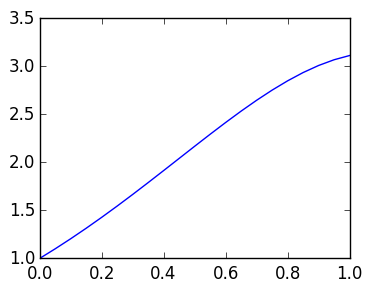## Forward Euler is unstable if the step size is too large¶

Consider the simple ODE

$$u' +\alpha u = 0, u(0)=1$$

which has the solution $e^{-\alpha x}$. The true solution decays exponentially. However, if we choose $h$ too large we get exponential increase:

In :
a=x->-30.
f=zeros   # zero RHS
c=1.

h=0.1
n=100

x=0.:h:n*h  # domain grid
r=x[1:end-1]  # range grid
B=[1 zeros(1,n)]  # initial condition
L=D(h,n) -  A_left(a,h,n)  # differential operator u' -a(x)*u

M=M_fe=[B; L]

w=M\[c;f(r)]

plot(x,w);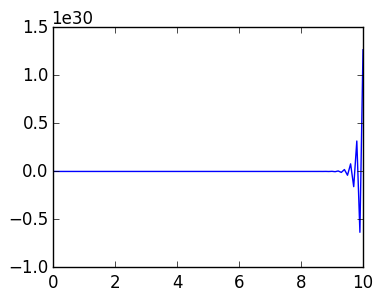# Backward Euler¶

To avoid this instability, we introduce backward Euler. The idea is to have the operators map to $x_1,\ldots,x_n$ instead of $x_0,\ldots,x_{n-1}$. That is to construct $D_n$, we approximate by trapezoids, differentiate exactly and evaluate at $x_1-0,\ldots,x_n-0$:

In :
f=x->cos(20cos(x))
n=4
h=1/n
x=linspace(0.,1.,n+1)
vals=f(x)

#differentiate and evaluate at k*h-0

dvals=diff(vals)*h
for k=1:n
plot([(k-1)*h,k*h],[dvals[k],dvals[k]])
end

scatter(x[2:n+1],dvals)

axis([0,1,-0.5,0.5]);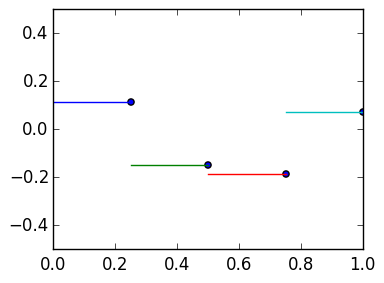The resulting discrete Derivative matrix has the same entries

$$D_n \triangleq {1 \over h} \begin{pmatrix} -1 & 1 \cr & -1 & 1 \cr &&\ddots & \ddots \cr &&& -1 & 1\end{pmatrix}$$

but now we interpret the map as

$$D_n : {\hbox{Values at } \atop x_0,\ldots,x_n} \rightarrow {\hbox{Values at } \atop x_1,\ldots,x_{n}}$$

This change affects the entries of $A_n$:

$$A_n \triangleq \begin{pmatrix} 0 & a(x_1) \cr && a(x_2) \cr &&&\ddots \cr &&&&a(x_{n}) \end{pmatrix},$$

where this now

$$A_n : {\hbox{Values at } \atop x_0,\ldots,x_n} \rightarrow {\hbox{Values at } \atop x_1,\ldots,x_{n}}.$$

We construct it as follows:

In :
function A_right(a::Function,h,n)
ret=zeros(n,n+1)
for k=1:n
ret[k,k+1]=a(k*h)
end
ret
end

Out:
A_right (generic function with 1 method)

$B_n$ is the same. We thus have a new operator which we solve using . A benefit is that the solution decays even for large $h$ when solving $u' + \alpha u = 0$:

In :
a=x->-30.
f=zeros   # zero RHS
c=1.

h=0.1
n=100

x=0.:h:n*h  # domain grid
r=x[2:end]  # range grid

B=[1 zeros(1,n)]  # initial condition
L=D(h,n) -  A_right(a,h,n)  # differential operator u' -a(x)*u

M=M_be=[B; L]

w=M\[c;f(r)]

plot(x,w);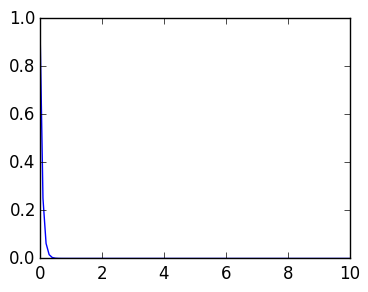In :
a=x->-30.
f=zeros   # zero RHS
c=1.

h=0.001
n=100

x=0.:h:n*h  # domain grid
r=x[2:end]  # range grid

B=[1 zeros(1,n)]  # initial condition
L=D(h,n) -  A_right(a,h,n)  # differential operator u' -a(x)*u

M=M_be=[B; L]

w=M\[c;f(r)]

norm(w - exp(-30.x),Inf)

Out:
0.00544995956956007
In :
M_be

Out:
101x101 Array{Float64,2}:
1.0      0.0      0.0      0.0  …      0.0      0.0      0.0     0.0
-1000.0   1030.0      0.0      0.0         0.0      0.0      0.0     0.0
0.0  -1000.0   1030.0      0.0         0.0      0.0      0.0     0.0
0.0      0.0  -1000.0   1030.0         0.0      0.0      0.0     0.0
0.0      0.0      0.0  -1000.0         0.0      0.0      0.0     0.0
0.0      0.0      0.0      0.0  …      0.0      0.0      0.0     0.0
0.0      0.0      0.0      0.0         0.0      0.0      0.0     0.0
0.0      0.0      0.0      0.0         0.0      0.0      0.0     0.0
0.0      0.0      0.0      0.0         0.0      0.0      0.0     0.0
0.0      0.0      0.0      0.0         0.0      0.0      0.0     0.0
0.0      0.0      0.0      0.0  …      0.0      0.0      0.0     0.0
0.0      0.0      0.0      0.0         0.0      0.0      0.0     0.0
0.0      0.0      0.0      0.0         0.0      0.0      0.0     0.0
⋮                               ⋱                                ⋮
0.0      0.0      0.0      0.0         0.0      0.0      0.0     0.0
0.0      0.0      0.0      0.0  …      0.0      0.0      0.0     0.0
0.0      0.0      0.0      0.0         0.0      0.0      0.0     0.0
0.0      0.0      0.0      0.0         0.0      0.0      0.0     0.0
0.0      0.0      0.0      0.0         0.0      0.0      0.0     0.0
0.0      0.0      0.0      0.0         0.0      0.0      0.0     0.0
0.0      0.0      0.0      0.0  …      0.0      0.0      0.0     0.0
0.0      0.0      0.0      0.0         0.0      0.0      0.0     0.0
0.0      0.0      0.0      0.0      1030.0      0.0      0.0     0.0
0.0      0.0      0.0      0.0     -1000.0   1030.0      0.0     0.0
0.0      0.0      0.0      0.0         0.0  -1000.0   1030.0     0.0
0.0      0.0      0.0      0.0  …      0.0      0.0  -1000.0  1030.0

Both Forward Euler and Backward Euler have the property that their discretizations converge when applying the operator:

In :
r_fe=x[1:end-1]
norm(M_fe*cos(x)  - [1.;(sin(r_fe) +30.*cos(r_fe))],Inf)

Out:
0.09983172171034482
In :
r_be=x[2:end]
norm(M_be*cos(x)  - [1.;(sin(r_be) +30.*cos(r_be))],Inf)

Out:
0.19916931461358445

The issue is that the norm of the inverse is very large for Forward Euler:

In :
norm(inv(M_be)),norm(inv(M_fe))

Out:
(4.168472905975071,1.4661943956636035e30)

# Midpoint rule¶

We now introduce one more method, where we evaluate at $x_{1/2},x_{3/2},\ldots,x_{n-1/2}$:

In :
f=x->cos(20cos(x))
n=4
h=1/n
x=linspace(0.,1.,n+1)
vals=f(x)

#differentiate and evaluate at k*h-0

dvals=diff(vals)*h
for k=1:n
plot([(k-1)*h,k*h],[dvals[k],dvals[k]])
end

scatter(h/2:h:(n-1/2)*h,dvals)

axis([0,1,-0.5,0.5]);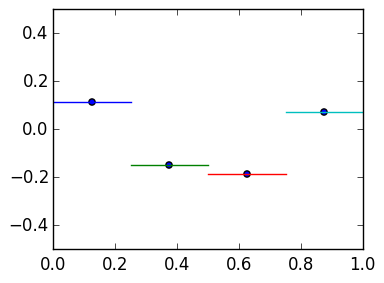$D_n$ again has the same entries, but now we interpret as

$$D_n : {\hbox{Values at } \atop x_0,\ldots,x_n} \rightarrow {\hbox{Values at } \atop x_{1/2},\ldots,x_{n-1/2}}$$

$A_n$ has changed, however: evaluating the trapezoidal approximation at the midpoint is equivalent to averaging the values, that is, the approximation at, say, $x_{1/2}$ is $(u_1 +u_0)/2$. We multiply by the true $a(x)$ evaluated at $x_{1/2}$ however to get $a(x_{1/2})(u_1 +u_0)/2$. Thus we have the $n \times n+1$ matrix:

$$A_n \triangleq {1 \over 2} \begin{pmatrix} a(x_{1/2}) & a(x_{1/2}) \cr &a(x_{3/2})& a(x_{3/2}) \cr &&\ddots&\ddots \cr &&&a(x_{n-1/2})&a(x_{n-1/2}) \end{pmatrix},$$
In :
function A_mid(a::Function,h,n)
ret=zeros(n,n+1)
for k=1:n
ret[k,k]=ret[k,k+1]=a((k-1/2)*h)/2
end
ret
end

Out:
A_mid (generic function with 1 method)

We use this to approximate:

In :
a=x->-30.
f=zeros   # zero RHS
c=1.

h=0.001
n=100

function M_mid(a,f,h,n)
x=0.:h:n*h  # domain grid
r=h/2:h:(n-1/2)*h

B=[1 zeros(1,n)]  # initial condition
L=D(h,n) -  A_mid(a,h,n)  # differential operator u' -a(x)*u

[B; L]
end

h=0.05
n=10
M=M_mid(a,f,h,n)
r=h/2:h:(n-1/2)*h

w=M\[c;f(r)]
plot(w)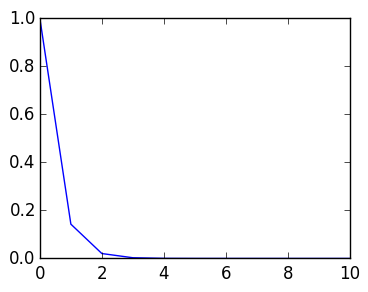Out:
1-element Array{Any,1}:
PyObject <matplotlib.lines.Line2D object at 0x318a6da10>

What we see is that it converges to the true solution much quicker, like $O(n^{-2})$ instead of $O(n^{-1})$:

In :
n=10000

h=1/n

M=M_mid(a,f,h,n)

x=0:h:n*h
r=h/2:h:(n-1/2)*h

norm(M*cos(x) - [c;(-sin(r)   + 30.cos(r))],Inf)  # goes down like 1/n^2

Out:
3.750169597083186e-8

We leave it as an exercise to show why combining

$$\|M_n^{-1}\|_\infty = O(1)$$

as $n\rightarrow \infty$ (that is, its bounded) with the fact that

$$\left\|M_n \begin{pmatrix} u(x_0)\cr\vdots\cr u(x_n)\end{pmatrix} - \begin{pmatrix} c \cr f(x_{1/2}) \cr \vdots \cr f(x_{n-1/2}) \end{pmatrix}\right\|_\infty = O(n^{-2})$$

is sufficient to prove that we converge like $O(n^{-2})$ to the true solution:

$$\left\|\begin{pmatrix} u(x_0)\cr\vdots\cr u(x_n)\end{pmatrix} - \begin{pmatrix} w_0\cr\vdots\cr w_n\end{pmatrix}\right\|_\infty = O(n^{-2})$$

This is a general principle in numerical analysis: Stability and Approximation imply Convergence.